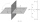# Circle - AG

Find the coordinates of circle and its diameter if its equation is:Result

x1 =  0
y1 =  2
D =  14

#### Solution:Leave us a comment of example and its solution (i.e. if it is still somewhat unclear...):

Showing 0 comments:Be the first to comment!#### To solve this example are needed these knowledge from mathematics:

For Basic calculations in analytic geometry is helpful line slope calculator. From coordinates of two points in the plane it calculate slope, normal and parametric line equation(s), slope, directional angle, direction vector, the length of segment, intersections the coordinate axes etc. Pythagorean theorem is the base for the right triangle calculator.

## Next similar examples:

1. Equation of circlefind an equation of the circle with indicated properties: a. center at (-3,5), diameter 20. b. center at origin and diameter 16.
2. CenterCalculate the coordinates of the circle center: ?
3. RT and circlesSolve right triangle if the radius of inscribed circle is r=9 and radius of circumscribed circle is R=23.
4. Circle chordDetermine the radius of the circle in which the chord 6 cm away from the center of the circle is 12 cm longer than the radius of the circle.
5. CircleWrite the equation of a circle that passes through the point [0,6] and touch the X-axis point [5,0]: ?
6. Circle annulusThere are 2 concentric circles in the figure. Chord of larger circle 10 cm long is tangent to the smaller circle. What are does annulus have?
7. Circle's chordsIn the circle there are two chord length 30 and 34 cm. The shorter one is from the center twice than longer chord. Determine the radius of the circle.
8. Chord - TS v2The radius of circle k measures 87 cm. Chord GH = 22 cm. What is TS?
9. The fenceI'm building a fence. Late is rounded up in semicircle. The tops of late in the field between the columns are to copy an imaginary circle. The tip of the first and last lath in the field is a circle whose radius is unknown. The length of the circle chord i
10. Parametric equationFind the parametric equation of a line with y-intercept (0,-4) and a slope of -2.
11. Add vectorGiven that P = (5, 8) and Q = (6, 9), find the component form and magnitude of vector PQ.
12. Symmetry by planeDetermine the coordinates of a image of point A (3, -4, -6) at a symmetry that is determined by the plane x-y-4z-13 = 0
13. Center of line segmentCalculate the distance of the point X [1,3] from the center of the line segment x = 2-6t, y = 1-4t ; t is .
14. Theorem proveWe want to prove the sentense: If the natural number n is divisible by six, then n is divisible by three. From what assumption we started?
15. GP membersThe geometric sequence has 10 members. The last two members are 2 and -1. Which member is -1/16?
16. Third memberDetermine the third member of the AP if a4=93, d=7.5.
17. Calculation of CNCalculate: ?